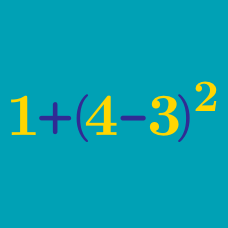Everyday Math

# Basic Arithmetic - Word Problems

There are $9$ students in Josh's class, and there are $6$ other classes of the same size in this school (and no other classes). What is the total number of students in this school?

Juan has $\41$ left after spending $\10$ on groceries. How much money (in $) did Juan have before going to the grocery store? In one game, Michael Jordan of the Chicago Bulls scored $30$ points from $9$ successful $2$-point shots and $x$ successful $3$-point shots. What is the value of $x$? An online music store charges$$15$ for each CD. There is also an additional shipping charge per order. If Sue paid a total of \$$195$ for ordering $12$ CDs, which of the following is the shipping charge for Sue's order?

Sudoku is a logic-based combinatorial number-placement puzzle. The objective is to fill a $9 \times 9$ grid with digits 1 to 9 such that each column, row and $3 \times 3$ box contains all the digits from 1 to 9.

If a Sudoku puzzle is filled correctly, what is the sum of all of the entries in the $9 \times 9$ grid?

×